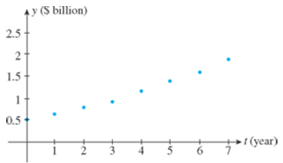Chapter 2.3, Problem 10TE

Chapter
Section
Textbook Problem

Web Conferencing Web conferencing is a big business, and it’s growing rapidly. The amount (in billions of dollars) spent on Web conferencing from the beginning of 2003 through 2010, and the scatter diagram for these data follow: Year 2003 2004 2005 2006 2007 2008 2009 2010 Amount 0.50 0.63 0.78 0.92 1.16 1.38 1.60 1.90a. Let t = 0 correspond to the beginning of 2003 and use QuadReg to find a second-degree polynomial regression model based 011 the given data. b. Plot the graph of the function f found in part (a) using the window [0, 7] × [0, 2]. c. Compute f(0), f(3), f(6), and f(7). Compare these values with the given data. Source: Gartner Dataquest.

(a)

To determine
The second degree polynomial regression model that best fits the data.

Explanation

Given:

The amount spent on web conferencing from 2003 to 2010.

Assume t=0 that corresponds to the year 2003, t=1 that corresponds to the year 2004, t=2 that corresponds to the year 2005, t=3 that corresponds to the year 2006, t=4 that corresponds to the year 2007, t=5 that corresponds to the year 2008, t=6 that corresponds to the year 2009 and t=7 that corresponds to the year 2010 as shown in table below.

 Year Number Ordered pair 2003 0.50 (0,0.50) 2004 0.63 (1,0.63) 2005 0.78 (2,0.78) 2006 0.92 (3,0.92) 2007 1.16 (4,1.16) 2008 1.38 (5,1.38) 2009 1.60 (6,1

(b)

To determine

To sketch: The graph of the polynomial f(t)=0.013t2+0.11t+0.503 .

(c)

To determine

To find: The values of the function at t=0,3,6and 7 and compare the values with the given data.

Still sussing out bartleby?

Check out a sample textbook solution.

See a sample solution

The Solution to Your Study Problems

Bartleby provides explanations to thousands of textbook problems written by our experts, many with advanced degrees!

Get Started

Evaluate the integral. 14t(1+t)dt

Calculus (MindTap Course List)

Use the guidelines of this section to sketch the curve. y=xx21

Single Variable Calculus: Early Transcendentals, Volume I

In problems 37-48, compute and simplify so that only positive exponents remain. 46.

Mathematical Applications for the Management, Life, and Social Sciences

With P and Q subsets of U as shown, shade ~PQ.

Elementary Geometry For College Students, 7e